## Applications of Newton’s Laws

Net force affects the motion, postion and/or shape of objects (some important and commonly used forces are friction, drag and deformation).

### Learning Objectives

Explain the effect of forces on an object’s motion and shape

### Key Takeaways

#### Key Points

• Friction is the force that resists relative motion between two surfaces sliding across each other. Friction converts kinetic energy into heat.
• Drag force is the force that resists motion of an object traveling through a fluid such as air or water. Drag force is proportional to the velocity of the object traveling.
• Deformation forces are forces caused by stretching or compressing a material. Some examples would be springs or elastics.

#### Key Terms

• kinetic energy: The energy possessed by an object because of its motion, equal to one half the mass of the body times the square of its velocity.

We know that a net force affects the motion, position and shape of an object. It is useful at this point to look at some particularly interesting and common forces that will provide further applications of Newton’s laws of motion. Specifically, we will discuss the forces of friction, air or liquid drag, and deformation.

### Friction

Friction is a force that resists movement between two surfaces sliding against each other. When surfaces in contact move relative to each other, the friction between the two surfaces converts kinetic energy into heat. This property can have a dramatic effect, as seen in the use of friction created by rubbing pieces of wood together to start a fire. Friction is not itself a fundamental force, but arises from fundamental electromagnetic forces between the charged particles constituting the two contacting surfaces.

### Drag

Another interesting force in everyday life is the force of drag on an object when it is moving in a fluid (either gas or liquid). You feel this drag force when you move your hand through water, or through the wind. Like friction, the force of drag is a force that resists motion. As we will discuss in later units, the drag force is proportional to the velocity of the object moving through it. We see an illustrated example of drag force in.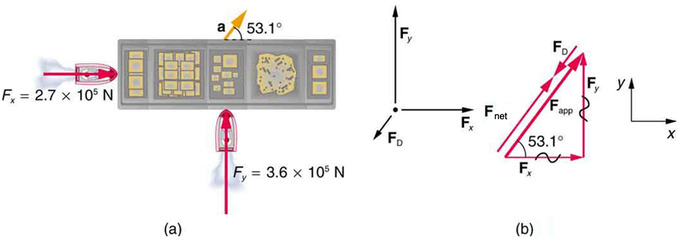Drag Force on a Barge: (a) A view from above of two tugboats pushing on a barge. (b) The free-body diagram for the ship contains only forces acting in the plane of the water. It omits the two vertical forces—the weight of the barge and the buoyant force of the water supporting it cancel and are not shown. Since the applied forces are perpendicular, the $\text{x}$– and $\text{y}$-axes are in the same direction as $\text{F}_\text{x}$ and $\text{F}_\text{y}$. The problem quickly becomes a one-dimensional problem along the direction of $\text{F}_{\text{app}}$, since friction is in the direction opposite to $\text{F}_{\text{app}}$.

### Deformation

We now move from consideration of forces that affect the motion of an object (such as friction and drag) to those that affect an object’s shape. If a bulldozer pushes a car into a wall, the car will not move but it will noticeably change shape. The change in shape of an object due to the application of a force is a deformation. Even very small forces are known to cause some deformation. For small deformations, two important characteristics are observed. First, the object returns to its original shape when the force is removed (that is, the deformation is elastic for small deformations). Second, the size of the deformation is proportional to the force.

## Friction: Kinetic

If two systems are in contact and moving relative to one another, then the friction between them is called kinetic friction.

### Learning Objectives

Explain the dynamics of energy for friction between two surfaces

### Key Takeaways

#### Key Points

• Kinetic (or dynamic) friction occurs when two objects are moving relative to each other and rub together (like a sled on the ground).
• The force of friction can be represented by an equation $\text{F}_{\text{friction}} = \mu \text{F}_\text{n}$where $\mu$ is the coefficient of friction and is a unitless number that represents the strength of the friction of the surface.
• Kinetic friction and static (stationary) friction use two different coefficients for the same material.

#### Key Terms

• kinetic energy: The energy possessed by an object because of its motion, equal to one half the mass of the body times the square of its velocity.

When surfaces in contact move relative to each other, the friction between the two surfaces converts kinetic energy into heat. This property can have dramatic consequences, as illustrated by the use of friction created by rubbing pieces of wood together to start a fire. Kinetic energy is converted to heat whenever motion with friction occurs, for example when a viscous fluid is stirred.

Kinetic Friction Introduction: Here, I’ll explain the microscopic justification of friction and what we can know about it. The coefficient of friction, too!

Kinetic (or dynamic) friction occurs when two objects are moving relative to each other and rub together; a sled on the ground would be a good example of kinetic friction.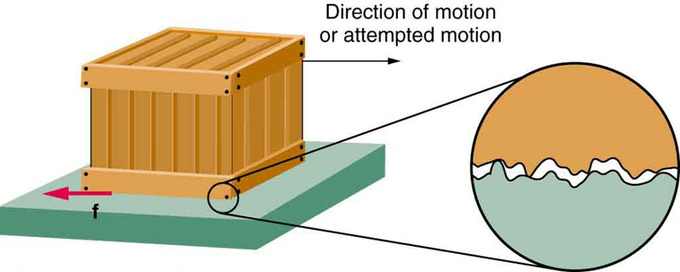Friction: Frictional forces always oppose motion or attempted motion between objects in contact. Friction arises in part because of the roughness of the surfaces in contact, as seen in the expanded view. In order for the object to move, it must rise to where the peaks can skip along the bottom surface. Thus, a force is required just to set the object in motion. Some of the peaks will be broken off, also requiring a force to maintain motion. Much of the friction is actually due to attractive forces between molecules making up the two objects, so that even perfectly smooth surfaces are not friction-free. Such adhesive forces also depend on the substances the surfaces are made of, explaining, for example, why rubber-soled shoes slip less than those with leather soles.

The force of friction is what slows an object sliding over a surface. This force is what makes the brakes on cars work or causes resistance when you slide your hand across a surface. The force of friction can be represented by an equation: $\text{F}_{\text{friction}} = \mu \text{F}_\text{n}$. In this equation $\mu$ is something called the coefficient of friction. This is a unitless number that represents the strength of the friction of the object. A very “grippy” surface like rubber might have a high coefficient of friction, whereas a slippery surface like ice has a much lower coefficient. $\text{F}_\text{n}$ is called the normal force and is the force of the surface pushing up on the object. In most cases on level ground, the normal force will be the equal and opposite of the object’s weight. In other words, it is the force that the surface must exert to keep the object from falling through.

The coefficient of kinetic friction is typically represented as $\mu_\text{k}$ and is usually less than the coefficient of static friction for the same materials.

## Friction: Static

Static friction is a type of friction that occurs to resist motion when two objects are at rest against each other.

### Learning Objectives

Demonstrate the relationship of maximum force of static friction

### Key Takeaways

#### Key Points

• Static friction is a force that acts to resist the start of motion. It is borne of macroscopic inconsistencies in the surfaces of materials in contact as well as intermolecular interactions between the materials, such as hydrogen bonding, Van der Waal’s interactions and electrostatic interactions.
• Static friction uses a different, usually higher, coefficient than kinetic friction does.
• The force of static friction is $\text{F}_{\text{fs}} = \mu_\text{s} \text{F}_\text{n}$. Where $\mu_\text{s}$ is the coefficient of static friction which varies by material and $\text{F}_\text{n}$ is the normal force.

#### Key Terms

• static: Fixed in place; having no motion.
• kinetic: Of or relating to motion
• friction: A force that resists the relative motion or tendency to such motion of two bodies in contact.

### Static Friction

Another type of frictional force is static friction, otherwise known as stiction. Like all friction, it acts to resist the motion of an object moving over a surface. Unlike kinetic friction, however, static friction acts to resist the start of motion.

Static Friction and some friction challenges: Here, I talk about sneaky ol’ static friction.

Static friction is friction between two objects that are not moving relative to each other. This frictional force is what prevents a parked car from sliding down a hill, for example. Before an object at rest on a surface can move, it must overcome the force of static friction.

Static friction originates from multiple sources. For any given material on another material of the same composition, friction will be greater as the material surfaces become rougher (consider sandpaper) on the macroscopic level. Additionally, intermolecular forces can greatly influence friction when two materials are put into contact. When surface area is below the micrometer range, Van der Waals’ forces, electrostatic interactions and hydrogen bonding can cause two materials to adhere to one another. A force is required to overcome these interactions and cause the surfaces to move across one another.

Like kinetic friction, the force of static friction is given by a coefficient multiplied by the normal force. The normal force is the force of the surface pushing up on the object, which is usually equal to the object’s weight. The coefficient of static friction is usually greater than the coefficient of kinetic friction and is usually represented by $\mu_\text{s}$.

Putting these elements together gives the maximum force of static friction as:

$\text{F}_{\text{s}} = \mu_\text{s}\text{F}_\text{n}$

In general, the force of static friction can be represented as:

$\text{F}_\text{s} \leq \mu_\text{s} \text{F}_\text{n}$

As with all frictional forces, the force of friction can never exceed the force applied. Thus the force of static friction will vary between 0 and $_\text{s}\text{F}_\text{n}$ depending on the strength of the applied force. Any force smaller than $_\text{s}\text{F}_\text{n}$ attempting to slide one surface over the other is opposed by a frictional force of equal magnitude and opposite direction. Any force larger than that overcomes the force of static friction and causes sliding to occur. The instant sliding occurs, static friction is no longer applicable—the friction between the two surfaces is then called kinetic friction.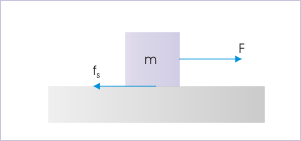Static Friction: To move a block at rest on a surface, a force must be applied which is great enough to overcome the force of static friction.

## Problem-Solving With Friction and Inclines

Combining motion on inclines with friction uses such concepts as equilibrium and contact force on an incline.

### Learning Objectives

Calculate the force of friction on an incline

### Key Takeaways

#### Key Points

• Motion on an incline is resisted by friction.
• The frictional force on an incline is dependent on the angle of the incline. $\text{F}_\text{f} = \mu \text{mg} \cos(\theta)$ is the maximum friction force on an incline.
• If the friction force is greater than or equal to the forces in the direction of motion, then the net force is 0 and the object is in equilibrium.

#### Key Terms

• friction: A force that resists the relative motion or tendency to such motion of two bodies in contact.
• incline: A slope.
• equilibrium: The state of a body at rest or in uniform motion, the resultant of all forces on which is zero.

### Contact Force on an Incline

The incline plane has two contact or interface surfaces. One is the incline surface, where the block is placed and the other is the base of the incline, which is in contact with the surface underneath. The motion of the block, therefore, may depend on the motion of the incline itself.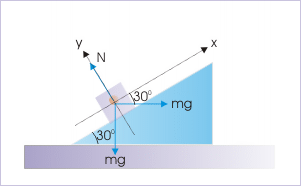Block and incline system: Forces on the block

When on an incline, calculating the force of friction is different than when the object is on a level surface. Recall that the force of friction depends on both the coefficient of friction and the normal force. $\text{F}_\text{f} = \mu \text{F}_\text{n}$ When on an incline with an angle $\theta$, the normal force becomes $\text{F}_\text{n}=\text{mg}\cos(\theta)$

As always, the frictional force resists motion. If the block is being pushed up the incline the friction force points down the incline. If the block is being pulled down the incline, the friction force will hold the block up.

### Equilibrium of Forces on an Incline

When not acted on by any other forces, only by gravity and friction, the frictional force will resist the tendency of the block to slide down the incline. If the frictional force is equal to the gravitational force the block will not slide down the incline. The block is said to be in equilibrium since the sum of the forces on it is 0.

Gravitational force down an incline is given by $\text{mg}\sin(\theta)$.

Where $\theta$ is the angle the incline makes with the horizontal. For the block to be in equilibrium, the maximum force of friction $\text{F}_\text{f} = \mu \text{mg}\cos(\theta)$ must be greater than or equal to $\text{F}_\text{G} = \text{mg}\sin(\theta)$. If the maximum frictional force is greater than the force of gravity, the sum of the forces is still 0. The force of friction can never exceed the other forces acting on it. The frictional forces only act to counter motion.

## Drag

The drag force is the resistive force felt by objects moving through fluids and is proportional to the square of the object’s speed.

### Learning Objectives

Relate the magnitude of drag force to the speed of an object

### Key Takeaways

#### Key Points

• Objects moving through a fluid feel a force which resists their motion. This force is known as the drag force.
• The drag force is proportional to the square of the velocity of the object relative to the fluid.
• The equation for drag is $\text{F}_\text{D} = \frac{1}{2} \text{C} \rho \text{A} \text{v}^2$. $\text{C}$ is a constant called the drag coefficient. $\rho$ is the density of the fluid. $\text{A}$ is the surface area in the direction of motion.

#### Key Terms

• fluid: Any substance which can flow with relative ease, tends to assume the shape of its container, and obeys Bernoulli’s principle; a liquid, gas or plasma.

Another interesting force in everyday life is the force of drag on an object when it is moving in a fluid (either a gas or a liquid). You feel the drag force when you move your hand through water. You might also feel it if you move your hand during a strong wind. The faster you move your hand, the harder it is to move. You feel a smaller drag force when you tilt your hand so only the side goes through the air—you have decreased the area of your hand that faces the direction of motion.

Like friction, the drag force always opposes the motion of an object. Unlike simple friction, the drag force is proportional to some function of the velocity of the object in that fluid. This functionality is complicated and depends upon the shape of the object, its size, its velocity, and the fluid it is in. Aerodynamic objects tend to have small surface areas and be designed to have low drag coefficients.

For most large objects such as bicyclists, cars, and baseballs not moving too slowly, the magnitude of the drag force $\text{F}_\text{D}$ is found to be proportional to the square of the speed of the object. We can write this relationship mathematically as $\text{F}_\text{D} \propto \text{v}^2$. When taking into account other factors, this relationship becomes $\text{F}_\text{D} = \frac{1}{\frac{2\text{C}}{rho \text{A} \text{v}^2}}$, where $\text{C}$ is known as the drag coefficient, a unit-less number that represents the aerodynamic properties of the object, $\text{A}$ is the cross-sectional area of the object which is facing the direction of motion, and $\rho$ is the density of the fluid the object is moving through.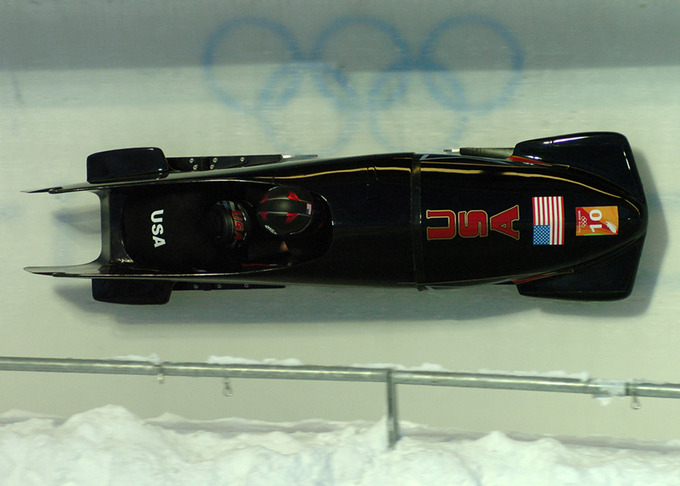Aerodynamic Shape: From racing cars to bobsled racers, aerodynamic shaping is crucial to achieving top speeds. Bobsleds are designed for speed. They are shaped like a bullet with tapered fins. (credit: U.S. Army, via Wikimedia Commons)

Retarding and Drag Forces: A brief look at retarding (drag) forces in physics, for students in introductory physics classes that use calculus. This video walks through a single scenario of an object experiencing a drag force where the drag force is proportional to the object’s velocity.

## Stress and Strain

The ratio of force to area $\frac{\text{F}}{\text{A}}$ is called stress and the ratio of change in length to length $\frac{\Delta \text{L}}{\text{L}}$ is called the strain.

### Learning Objectives

Explain how forces affects the shape of an object

### Key Takeaways

#### Key Points

• The ratio of force to area $\frac{\text{F}}{\text{A}}$ is called stress and the ratio of change in length to length $\frac{\Delta \text{L}}{\text{L}}$ is called the strain.
• Stress and strain are related to each other by a constant called Young’s Modulus or the elastic modulus which varies depending on the material. Using Young’s Modulus the relation between stress and strain is given by: $\text{stress} = \text{Y}\cdot \text{strain}$.
• A material with a high elastic modulus is said to have high tensile strength. Such materials are very resistant to being stretched and require a large amount of force to deform a small amount.

#### Key Terms

• strain: The amount by which a material deforms under stress or force, given as a ratio of the deformation to the initial dimension of the material and typically symbolized by $\epsilon$ is termed the engineering strain. The true strain is defined as the natural logarithm of the ratio of the final dimension to the initial dimension.
• stress: The internal distribution of force per unit area (pressure) within a body reacting to applied forces which causes strain or deformation and is typically symbolized by $\sigma$.

We now move from consideration of forces that affect the motion of an object (such as friction and drag) to those that affect an object’s shape. If a bulldozer pushes a car into a wall, the car will not move past the wall but it will noticeably change shape. A change in shape due to the application of a force is a deformation. Even very small forces are known to cause some deformation. For small deformations, two important characteristics are observed. First, the object returns to its original shape when the force is removed—that is, the deformation is elastic for small deformations. Second, the size of the deformation is proportional to the force—that is, for small deformations, Hooke’s law is obeyed. In equation form, Hooke’s law is given by $\text{F} = \text{k} \cdot \Delta \text{L}$ where $\Delta \text{L}$ is the change in length and $\text{k}$ is a constant which depends on the material properties of the object.

Deformations come in several types: changes in length (tension and compression), sideways shear (stress), and changes in volume.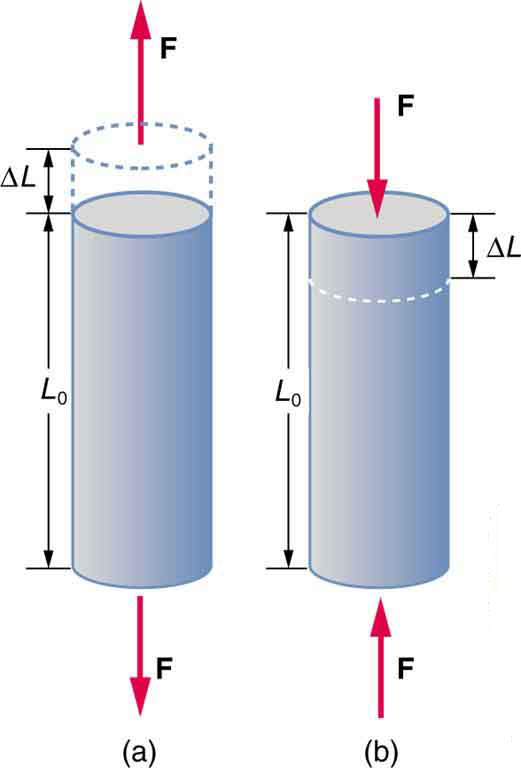Tension/Compression: Tension: The rod is stretched a length $\Delta \text{L}$ when a force is applied parallel to its length. (b) Compression: The same rod is compressed by forces with the same magnitude in the opposite direction. For very small deformations and uniform materials, $\Delta \text{L}$ is approximately the same for the same magnitude of tension or compression. For larger deformations, the cross-sectional area changes as the rod is compressed or stretched.

The ratio of force to area $\frac{\text{F}}{\text{A}}$ is called stress and the ratio of change in length to length $\frac{\Delta \text{L}}{\text{L}}$ is called the strain.

Stress and strain are related to each other by a constant called Young’s Modulus or the elastic modulus which varies depending on the material. Using Young’s Modulus the relation between stress and strain is given by: $\text{stress} = \text{Y}\cdot\text{strain}$.

A material with a high elastic modulus is said to have high tensile strength. Such materials are very resistant to being stretched and require a large amount of force to deform a small amount.

## Translational Equilibrium

An object is said to be in equilibrium when there is no external net force acting on it.

### Learning Objectives

Assess the role each type of equilibrium plays in mechanical devices

### Key Takeaways

#### Key Points

• When there is no external net force on an object, the object is said to be in equilibrium.
• When an object is in equilibrium, it does not accelerate. If it had a velocity, the velocity remains constant; if it was at rest, it remains at rest.
• An equilibrium in motion is known as dynamic equilibrium; an equilibrium at rest is a static equilibrium.

#### Key Terms

• dynamic: Changing; active; in motion.
• torque: A rotational or twisting effect of a force; (SI unit newton-meter or Nm; imperial unit foot-pound or ft-lb)
• static: Fixed in place; having no motion.

We are surrounded by great engineering architectures and mechanical devices, which are at rest in the frame of reference of Earth. A large part of engineering creations are static objects. Yet we also seek equilibrium of moving objects like that of floating ship, airplane cruising at high speed, and such other moving mechanical devices. In both cases – static or dynamic – net external forces and torques are zero.

A body is said to be in mechanical equilibrium when net external force is equal to zero and net external torque is also zero. Mathematically,

$\Sigma \vec {\text{F}}_{\text{ext}} = 0$ and $\Sigma \vec \tau_{\text{ext}} = 0$.

Since there is no net force on the object, the object does not accelerate. This implies two types of possible equilibrium. The first type, where all particles in the system are at rest and do have not velocity, is known as static equilibrium. In the second type, the object has a velocity, but since there are no net forces acting on it, the velocity remains constant. In the second case, the particle is said to be in dynamic equilibrium. Static or dynamic, these kinds of equilibrium can be categorized as translational equilibrium.

Examples of translational equilibrium are all around us. A book resting on a table is pushing down on the table with the force of its weight. The table, in turn, is pushing back on the book, keeping the book from falling through the table. Since neither the table nor the book are moving, this is an example of static equilibrium. The force of gravity on the book is perfectly counteracted by the force of the table pushing on it.Forces Acting on an Object at Rest: A force diagram showing the forces acting on an object at rest on a surface. Notice that the amount of force that the table is pushing upward on the object (the N vector) is equal to the downward force of the object’s weight (shown here as mg, as weight is equal to the object’s mass multiplied by the acceleration due to gravity): because these forces are equal, the object is in a state of equilibrium (all the forces acting on it balance to zero).

An example of dynamic (or mechanical) equilibrium is an object sliding down a wedge. The force of gravity pulls the object down the wedge, but it is counteracted by the force of friction between the wedge and the object. If the force of friction is equal to the force of gravity, the object will proceed at a constant velocity.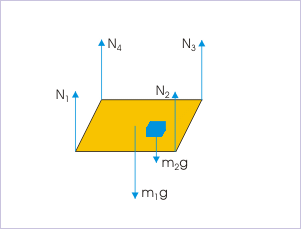Forces on a Table: These six forces are in equilibrium. The four forces of the table leg counteract the force of the table and the object pushing on them.

## Connected Objects

Forces can be transferred from one object to another through connections.

### Learning Objectives

Analyze the affect a rigid connection has on the movement of objects

### Key Takeaways

#### Key Points

• If two objects are connected, a force on one has an effect on the other.
• Connections can often be approximated as completely rigid. In completely rigid cases, the connection does not deform and the force is transferred instantaneously.
• Tension is the force of a rope or cable or other connector on the object it is connected to. It is one way force is transferred between objects.

#### Key Terms

• rigid: Stiff, rather than flexible.

The physics of connected objects is very similar to physics of simple objects. There are a variety of ways objects can be connected to each other, and a corresponding variety of mathematical ways to model such connections.

The simplest form of connection is a perfectly rigid connection. If two objects are connected by a perfectly rigid connector then they may be thought of as the same object. Perfectly rigid connectors cannot stretch nor deform, and transfer forces instantaneously from one side of the connection to the other. For example, given two blocks (both of mass 1 kg) connected by a perfectly rigid bar, if the first block is pulled with a force of 1 Newton, then both blocks will accelerate at the same time and the same acceleration. In this case the acceleration is $\frac{1}{2} \text{m/s}^2$ —the same as if a force of mass 2 kg is exerted on one object. Thus it can be said that a perfectly rigid connection makes two objects into one large object. Of course, perfectly rigid connections do not exist in nature. Some deformation will always exist in any object as force travels along it. However, many materials are sufficiently rigid, so that using the perfectly rigid approximation is useful for simplicity’s sake.

One can think of the force transferring through the connection by means of the “tension” force. Tension is the pulling force exerted by a string, chain, or similar connector on another object. If two objects are connected by a string, a force exerted on one is balanced by a tension force in the string which pulls on the other. Of course, if the tension force is greater than the rope can withstand, the rope will break.Tension Forces: The forces involved in supporting a ball by a rope. Tension is the force of the rope on the scaffold, the force of the rope on the ball, and the balanced forces acting on and produced by segments of the rope.

## Circular Motion

An object in circular motion undergoes acceleration due to centripetal force in the direction of the center of rotation.

### Learning Objectives

Develop an understanding of uniform circular motion as an indicator for net external force

### Key Takeaways

#### Key Points

• An object that is undergoing circular motion has a velocity vector that is constantly changing direction.
• The force that is needed to maintain circular motion points toward the center of the circular path. It is therefore known as the centripetal force.
• The velocity of an object in circular motion is always tangent to the circle, and the centripetal force is always perpendicular to the velocity.

#### Key Terms

• tangent: a straight line touching a curve at a single point without crossing it at that point
• perpendicular: at or forming a right angle (to).

Uniform circular motion describes the motion of an object along a circle or a circular arc at constant speed. It is the basic form of rotational motion in the same way that uniform linear motion is the basic form of translational motion. However, the two types of motion are different with respect to the force required to maintain the motion.

Let us consider Newton’s first law of motion. It states that an object will maintain a constant velocity unless a net external force is applied. Therefore, uniform linear motion indicates the absence of a net external force. On the other hand, uniform circular motion requires that the velocity vector of an object constantly change direction. Since the velocity vector of the object is changing, an acceleration is occurring. Therefore, uniform circular motion indicates the presence of a net external force.

In uniform circular motion, the force is always perpendicular to the direction of the velocity. Since the direction of the velocity is continuously changing, the direction of the force must be as well.

The direction of the velocity along the circular trajectory is tangential. The perpendicular direction to the circular trajectory is, therefore, the radial direction. Therefore, the force (and therefore the acceleration) in uniform direction motion is in the radial direction. For this reason, acceleration in uniform circular motion is recognized to “seek the center” — i.e., centripetal force.

The equation for the acceleration $\text{a}$ required to sustain uniform circular motion is:

$\displaystyle \text{a} =\frac{\text{v}^2}{\text{r}}$

where $\text{m}$ is the mass of the object, $\text{v}$ is the velocity of the object, and $\text{r}$ is the radius of the circle. Consequently, the net external force $\text{F}_{\text{net}}$ required to sustain circular motion is:

$\displaystyle \text{F}_{\text{net}}=\frac{\text{m}\cdot \text{v}^2}{\text{r}}$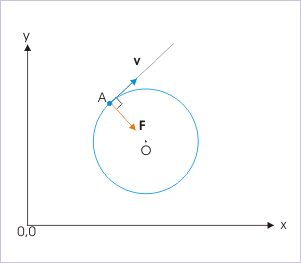Uniform Circular Motion: In uniform circular motion, the centripetal force is perpendicular to the velocity. The centripetal force points toward the center of the circle, keeping the object on the circular track.